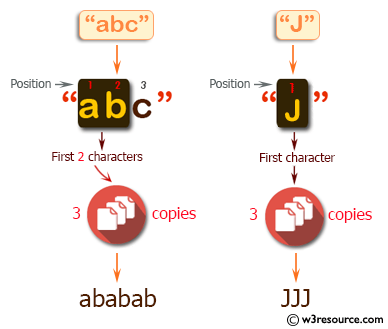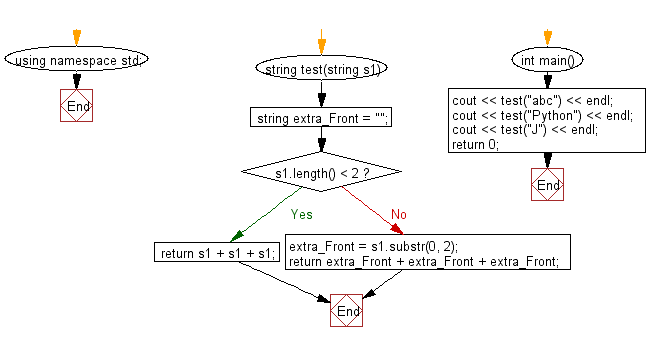﻿ C++ : String using 3 copies of the first 2 characters# C++ Exercises: Create a new string using 3 copies of the first 2 characters of a given string

## C++ Basic Algorithm: Exercise-77 with Solution

Write a C++ program to create a new string using 3 copies of the first 2 characters of a given string. If the length of the given string is less than 2 use the whole string.

Sample Solution:

C++ Code :

``````#include <iostream>
using namespace std;

string test(string s1)
{
string extra_Front = "";

if (s1.length() < 2)
{
return s1 + s1 + s1;
}
else
{
extra_Front = s1.substr(0, 2);
return extra_Front + extra_Front + extra_Front;
}
}

int main()
{
cout << test("abc") << endl;
cout << test("Python") << endl;
cout << test("J") << endl;
return 0;
}
``````

Sample Output:

```ababab
PyPyPy
JJJ
```

Pictorial Presentation:Flowchart:C++ Code Editor:

Contribute your code and comments through Disqus.

What is the difficulty level of this exercise?

﻿